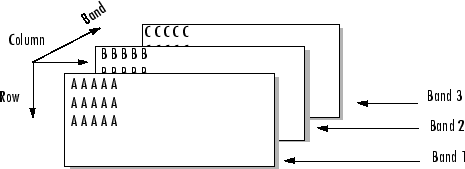# multibandwrite

## 语法

```multibandwrite(data,filename,interleave) multibandwrite(data,filename,interleave,start,totalsize) multibandwrite(...,param,value...) ```

## 说明

`multibandwrite(data,filename,interleave)` 将二维或三维数字或逻辑数组 `data` 写入 `filename` 所指定的二进制文件。`filename` 指定为字符向量或字符串标量。`data` 第三维的长度决定了写入文件的条带数。条带以 `interleave` 指定的格式写入文件。关于该参数的详细信息，请参阅交叉方法

`multibandwrite(data,filename,interleave,start,totalsize)``data` 按块写入二进制文件 `filename`。在此语法中，`data` 是完整数据集的子集。

`start` 是指定开始写入数据位置的 1×3 数组 `[firstrow firstcolumn firstband]``firstrow``firstcolumn` 指定左上方图像像素的位置。`firstband` 给出要写入的第一个条带的索引。例如，`data(I,J,K)` 包含了第 `(firstband+K-1)` 个条带中 [`firstrow+I-1, firstcolumn+J-1]` 位置像素的数据。

`totalsize` 是一个 1×3 数组，`[totalrows,totalcolumns,totalbands]`，它指定要写入文件的数据的完整三维大小。

`multibandwrite(...,param,value...)` 根据指定的可选参数/值对将多条带数据写入文件。

`'precision'`

`'offset'`

`'machfmt'`

`'fillvalue'`

## 交叉方法

`interleave` 是指定 `multibandwrite` 在将数据写入文件时如何交错条带的字符向量或字符串标量。如果 `data` 是二维的，`multibandwrite` 会忽略 `interleave` 参数。下表列出了支持的方法并用多条带文件示例对每种方法进行了解释。`'bil'`

```AAAAABBBBBCCCCC AAAAABBBBBCCCCC AAAAABBBBBCCCCC ```

`'bip'`

```ABCABCABCABCABC... ```

`'bsq'`

```AAAAA AAAAA AAAAA BBBBB BBBBB BBBBB CCCCC CCCCC CCCCC ```

## 示例

### 示例 1

```data = reshape(uint16(1:600), [10 20 3]); multibandwrite(data,'data.bil','bil'); ```

### 示例 2

```numBands = 1; dataDims = [1024 1024 numBands]; data = reshape(uint32(1:(1024 * 1024 * numBands)), dataDims); for band = 1:numBands for row = 1:2 for col = 1:2 subsetRows = ((row - 1) * 512 + 1):(row * 512); subsetCols = ((col - 1) * 512 + 1):(col * 512); upperLeft = [subsetRows(1), subsetCols(1), band]; multibandwrite(data(subsetRows, subsetCols, band), ... 'banddata.bsq', 'bsq', upperLeft, dataDims); end end end ```